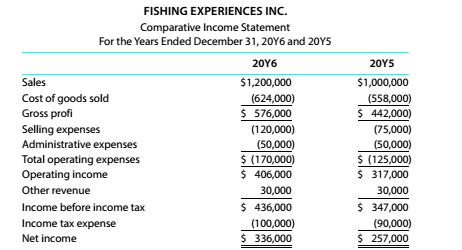Chapter 9, Problem 9.2.1P

Chapter
Section
Textbook Problem

Vertical analysis for income statement For 20Y6, Fishing Experiences Inc. initialed a sales promotion campaign that included the expenditure of an additional \$45,000 for advertising. At the end of the year, Colt Schultz. the president, is presented with the following condensed comparative income statement:Instructions Prepare a comparative income statement for the two-year period, presenting a vertical analysis of each item in relationship to sales for each of the years.

To determine

Concept Introduction:

Vertical Analysis:

Vertical Analysis is used to analyze the % of items in statement for a particular period as the % of total amount. Vertical Analysis is done for a particular period for different items. For example, analysis of % of current assets as % of Total assets for a particular year is done using the Vertical analysis.

To Prepare:

A comparative income statement using Vertical analysis.

Explanation

A comparative income statement using Vertical analysis is prepared as follows:

 Vertical Analysis of Income Statement 20X6 20Y5 Amount % Amount % A (A/1200000) B (B/1000000) Sales \$ 1,200,000 100.00% \$ 1,000,000 100.00% Cost of Goods Sold \$ (624,000) -52.00% \$ (558,000) -55.80% Gross Profit \$ 576,000 48.00% \$ 442,000 44.20% Selling expenses \$ (120,000) -10...

Still sussing out bartleby?

Check out a sample textbook solution.

See a sample solution

The Solution to Your Study Problems

Bartleby provides explanations to thousands of textbook problems written by our experts, many with advanced degrees!

Get Started